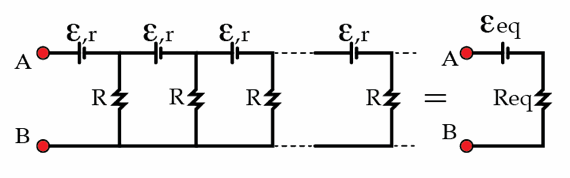# A very long circuit

A large number of AA batteries with emf $\mathcal{E}=1.5\, \mbox{V}$ and resistors with resistance $R$ form an infinite circuit, as shown in the figure. The internal resistance of the batteries is $r$. Thevenin's theorem tells us that this infinite circuit can be replaced by a single source with emf $\mathcal{E}_\text{eq}$, connected in series with an equivalent resistor $R_\text{eq}$. Determine the equivalent emf $\mathcal{E}_\text{eq}$ in volts if $\frac{r}{R}=\frac{1}{2}.$×